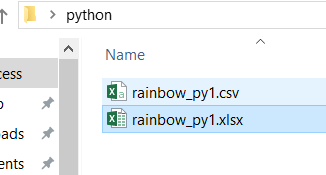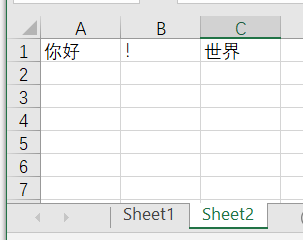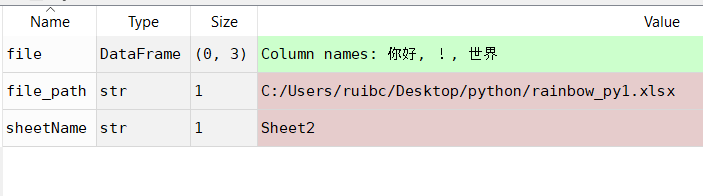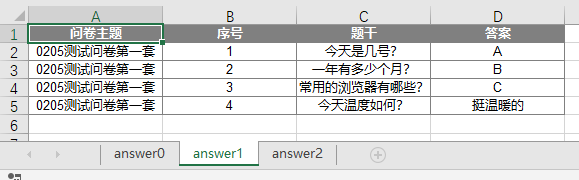• excel根据某个sheet中的第一列关键字不通拆分为多个sheet（第一列已排序的情况）。excel中把多个sheet合并为一个sheet的脚本
• labview自带的Excel库无删除sheet删除行列操作，本资源重新封装了接口，可以删除指定sheet表和指定行列内容，保证可用
• 自己写的代码，合并多个Excel中所有sheet内容，操作简单，注释明确，可灵活修改配置。。。。自己写的代码，合并多个Excel中所有sheet内容，操作简单，注释明确，可灵活修改配置
• 指定excelsheet另存为excel输出到指定的文件夹。 可以快速按固定的模版格式产生独立数据的报表。
• pandas 读取excel 指定sheet 1，准备excel 文件 文件2内容 2，python 代码 import pandas as pd ; #文件路径 file_path='C:/Users/ruibc/Desktop/python/rainbow_py1.xlsx' #读取sheet的名字 ...
python 读取excel 指定sheet
1，准备excel 文件文件2内容2，python 代码
   import pandas  as pd ;
#文件路径
file_path='C:/Users/ruibc/Desktop/python/rainbow_py1.xlsx'
#读取sheet的名字
sheetName='Sheet1'
file展开全文python pandas
• 如题,代码如下,执行后3个sheet原封不动的在那儿并未成功删除掉第一个sheet, 求助,帮忙寻找解决方法  '?获得Sheet的值.查看获得Sheet值得方法 ' '========================================= 'myString = ...vbscript
• poi无法直接获取excelsheet页打印页数，调用vbs脚本获取excelsheet页打印预览总页数。
• 可以将ExcelSheet的列表显示出来，并可以单击打开指定Sheet页面
• 修改excel.sheet12独立窗口故障.reg 修改excel.sheet12独立窗口故障.reg 修改excel.sheet12独立窗口故障.reg 修改excel.sheet12独立窗口故障.reg 修改excel.sheet12独立窗口故障.reg 修改excel.sheet12独立窗口故障....
• 提取excel工作表中多个sheet相同的单元格数据，并以列的形式存储在新的sheet
• Python excel不同sheet之间复制粘贴单元格含格式。代码中行和列都用数字表示，例如A 即 1
• 这个压缩包里边宏保存的时候丢掉了，我另外再传一个，见另一个资源... Excel vba 移动当前目录下边的excelSheet表到另一个新的excel表格，设计到文件创建 移动 删除 重命名等很多实用功能，备注很详细。适合初学者。
• 代码如下： import pandas as pd writer = pd.ExcelWriter(‘your_path.xlsx’) df1 = pd.DataFrame() ...df1.to_excel(writer, sheet_name=‘df_1’) df2.to_excel(writer, sheet_name=‘df_2’) writ...
代码如下： import pandas as pd
writer = pd.ExcelWriter(‘your_path.xlsx’)
df1 = pd.DataFrame() df2 = pd.DataFrame()
df1.to_excel(writer, sheet_name=‘df_1’) df2.to_excel(writer, sheet_name=‘df_2’) writer.save()
展开全文Python
• 说明：1和2方法在进行保存前会情况excelsheet页，及进行to_excel()时先清空表格的sheet页。2可以有多个sheet页 3会在原有excelsheet页后添加sheet页，不清空原有sheet页。 下面用例子说明： 1、import pandas...
1、直接使用df的to_excel()方法直接保存
2、使用ExcelWriter对象调用to_excel()方法进行保存
3、使用ExcelWriter 和openpyxl库进行excel操作
说明：1和2方法在进行保存前会情况excel的sheet页，及进行to_excel()时先清空表格的sheet页。2可以有多个sheet页
3会在原有excel的sheet页后添加sheet页，不清空原有sheet页。
下面用例子说明：
1、import pandas as pd
df = pd.DataFrame({'A':[1,2,3,4],'B':[11,12,13,14]}) #定义一个df对象
df.to_excel('test.xlsx',index=False) #将df对象保存为excel文件，不需要index

2 import pandas as pd
writer = pd.ExcelWriter('test.xlsx')
df = pd.DataFrame({'A':[1,2,3,4],'B':[11,12,13,14]})
df.to_excel(writer, sheet_name='test1')
df.to_excel(writer,sheet_name='test2')
writer.save()
writer.close()
说明：上面会保存2个sheet页，无论原test.xlsx文件是否有内容，或是否有test.xlsx文件，

3 import openpyxl
import pandas as pd
writer = pd.ExcelWriter('test.xlsx')
writer.book = wb
df1 = pd.DataFrame() #定义一个空df对象
df2 = pd.DataFrame({'A':[1,2,3,4],'B':[11,12,13,14]})
df1.to_excel(writer,sheet_name='test1', index=False)
df2.to_excel(writer,sheet_name='test2', index=False)
writer.save()
writer.close()


展开全文• 一般的Excel导出是按单个sheet的最大行数（默认65536 ）为基数，超过这个基数后再分一个sheet。 对数据的处理以 List<List<T>>格式操作的 简单来说就是多取一层list也就多一层循环,主要方法有以下两个。...
先交代下业务背景，做的是问卷调研的答卷导出，每一个人答卷设置一个sheet，
一般的Excel导出是按单个sheet的最大行数（默认65536 ）为基数，超过这个基数后再分一个sheet。
对数据的处理以 List<List<T>>格式操作的 简单来说就是多取一层list也就多一层循环,主要方法有以下两个。

	//填充excel
public void fillExcelDataExam(int index, Row row,int getList)
{
//lists --> List<List<T>>
List<T> ts = lists.get(getList);
int startNo = index * sheetSize;
int endNo = Math.min(startNo + sheetSize, ts.size());
for (int i = startNo; i < endNo; i++)
{
row = sheet.createRow(i + 1 - startNo);
// 得到导出对象.
T vo = (T) ts.get(i);
int column = 0;
for (Object[] os : fields)
{
Field field = (Field) os;
Excel excel = (Excel) os;
// 设置实体类私有属性可访问
field.setAccessible(true);
}
}
}
/**
* 对list数据源将其里面的数据导入到excel表单
*
* @return 结果
*/
public AjaxResult exportExcelExam()
{
OutputStream out = null;
try
{
for(int y = 0; y < lists.size(); y ++){
List<T> ts = lists.get(y);
// 取出一共有多少个sheet.
double sheetNo = Math.ceil(ts.size() / sheetSize);
for (int index = 0; index <= sheetNo; index++)
{
createSheet(lists.size(), y);

// 产生一行
Row row = sheet.createRow(0);
int column = 0;
// 写入各个字段的列头名称
for (Object[] os : fields)
{
Excel excel = (Excel) os;
this.createCell(excel, row, column++);
}
if (Type.EXPORT.equals(type))
{
fillExcelDataExam(index, row, y);
}
}
}

String filename = encodingFilename(sheetName);
out = new FileOutputStream(getAbsoluteFile(filename));
wb.write(out);
return AjaxResult.success(filename);
}
catch (Exception e)
{
log.error("导出Excel异常{}", e.getMessage());
}
finally
{
if (wb != null)
{
try
{
wb.close();
}
catch (IOException e1)
{
e1.printStackTrace();
}
}
if (out != null)
{
try
{
out.close();
}
catch (IOException e1)
{
e1.printStackTrace();
}
}
}
}

结果`
展开全文java spring
• ## Excel获取Sheet表名

千次阅读 热门讨论 2016-12-11 23:46:50
背景：最近在做Excel的导入导出功能，收获了很多的知识，首先采用数据库链接方式，使用OLEDB链接接口访问Excel表中的数据。 链接字符串： excel 2000 ~ 2003的 OleDb 连接串的格式如下： Provider=Microsoft.Jet....
• 而DataFrame生成excel中的sheet表，以及在excel中增加sheet表，在数据分 析中也经常用到。这里以一个简单例子介绍这两个功能的用法，以备不时之需。 例子: #!/usr/bin/env python # -*- codinpython pandas
• ## C#之excel新增sheet

千次阅读 2019-12-17 21:18:29
小伙伴们在用C#操作excel的时候，可能有需要新增sheet的需求，那么如何实现呢？ 二、思路 通过Epplus组件提供的ExcelPackage.Workbook.Worksheets.Add的添加方法来实现 1.组件：Epplus epplus组件的安装使用参考 在...
• 自动生成excelsheet目录导航： 1、alt+f11 2、插入-模版 Sub mulu() On Error GoTo Tuichu Dim i As Integer Dim ShtCount As Integer Dim SelectionCell As Range ShtCount = Worksheets.Count If ShtCount = 0 ...
• C# 怎么把Excel1的Sheet1 拷贝到 Excel2的Sheet1后面 备注：不是用一个Application打开两个Excel的方法，而是在两个ExcelSheet间直接拷贝操作   在C#中用代码实现上述操作：   ...c# null
• 读写excel数据类 ''' def __init__(self): self.wb = Workbook() self.tables_names = [] def readexcel(self): excel_datas = [] wb = load_workbook(read_excel_name) # ws = wb.acti
• asp.net实现数据导入导出到Excel中多Sheet的情况导出
• 最近需要使用POI操作Excel，有好多同一类型的sheet，需要复制操作，网上找了一下，居然都是逐行逐单元格操作的，我的天，这种操作实在有损我们程序员的英名，于是我就查了POI的所有操作sheet的方法，终于找到了...poi clone
• 今天业务说导出一个多sheet的功能报错了： java.lang.RuntimeException: 导出数据出现异常：The workbook already contains a sheet named 'MOLU6924723-1-GLE-(162-|-DA6CB)' 。。。。。。 看错误提示是...
• 本文描述了怎么利用MAV方法实现复杂的excel视图...